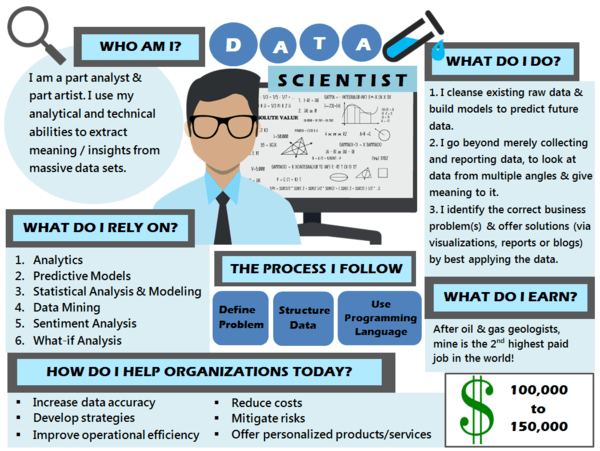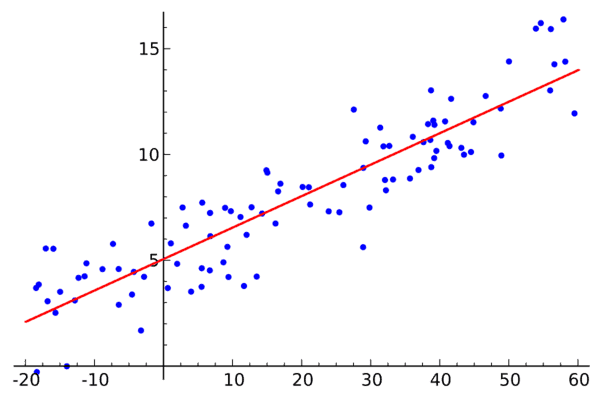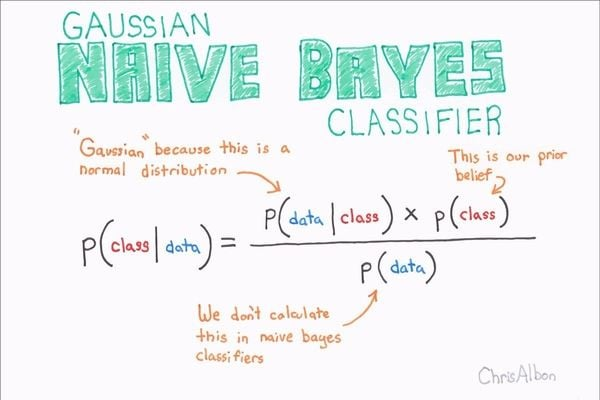﻿ Every Data Scientist Should Know These 10 Algorithms

# 10 Algorithms Every Data Scientist Should Know

In our world today, every task is being automated. Gone are the days when you had to walk for twenty days or a ride a horse for miles to get to a town or even do manual work such as carrying heavy logs. With our powerful minds, we have our work easier and much more efficient.

We have created Machine Learning Algorithms that enable machines to check our medical condition, play with us and even get smarter. We are living in an era where there is a rapid advancement in technology, and now we can predict what can happen in the future.In recent years, data scientists have designed and created sophisticated machines that execute advanced tasks easily and proficiently. And the results are just amazing! Therefore, learning these important aspects of algorithms will improve your skills about Machine Learning.

Here are the ten Algorithms every data scientist such as you should know today so that our future can be brighter.

Decision tree

A decision tree is an algorithm designed by answering either yes or no to questions with certain parameters. It is among the simplest ways of producing wonderfully defined algorithms. It eliminates over concentration and creation of large trees which is unnecessary in creating predictive algorithms. It works best when used to classify continuous dependent and categorical variables.

Linear regression

How would you arrange random logs of wood in order of their weight without actually weighing each log? You could gauge the weight of each log by just looking at it. And this is what linear regression is all about. It is about using visual analysis and arranging the parameters in order. In the end, a relationship is created between dependent and independent variables just by putting them on a line. The line is called a regression line and the equation represented by it is Y = a * X + bWhere Y is the dependent variable, a is the slope, X is the Independent variable and b is the intercept.

Logistic regression

Logistic regression has been used for a long time in estimating discrete values say binary values such zero and one from a group of independent variables. It enables you to predict the probability of an occurrence by feeding data into a logic function which is also known as a logit regression. Some of the methods used to improve logistic regression include eliminating features, including interaction terms, using non-linear models and regulating techniques.

Support Vector Machine

The support vector machine is a method used to classify how you plot data as points in x-dimensional space and x here represents the number of features you have. The value of a particular feature is combined with a particular coordinate thus making it easier for you classify your data. Lines known as classifiers are also used to split data and help you plot and create a graph.

Naïve Bayes

Naïve Bayes classifier works on the assumption that when a particular feature in a class is present, it is unrelated to the presence of another feature. If the features are related to each other, this algorithm will classify and consider all properties or variables independently when devising the probability of a certain outcome.A Naïve Bayes algorithm is easy to design and build for huge data. It is simple, practical and data scientists know that it outperforms very sophisticated methods of classification.

K Nearest Neighbors

K Nearest Neighbors can be easily understood by this example. If I want to know you better or to get more information about you, I can talk to your family, friends, and workmates about you.

This method can be used to classify and solve regression puzzles. In the Data Industry, it is used by many to solve classification puzzles and problems. It is an efficient algorithm that saves all cases available and classifies cases that are new thus taking the majority vote of its neighbors, in this case, k. Now the case is allocated to the class with highest similarity level. And a distance function is responsible for performing this operation.

Though this algorithm always works, it is expensive to compute, information acquired needs to be processed, and you should normalize the variables to avoid biases.

Random Forest

A Random Forest is simply a collection of decision trees. For you to classify an object or variable about its attributes, you need to classify each tree and the votes of each tree of that particular class. The forest then chooses a particular classification with the highest votes leaving all other trees in the forest. Every tree can be planted using the following procedure. (1) If X represents the number of cases in a set X, then a section of X cases is taken randomly.

The sample will then act as a training set to grow the trees. (2) In a case where there are Y variables to input then a number y <. (3) Every tree is grown to its full potential. No pruning is done.

K- Means

This algorithm is unsupervised and can solve clustering puzzles. Sets of data are classified in a particular quantity or number of clusters (in this case we will call it X) in a way that the data points in a cluster are heterogeneous and homogeneous from the information acquired from all clusters. How does K for clusters?

The K algorithm picks the number of points k known as centroids for a particular cluster. Every data point then creates a cluster with the centroids they are closest to that is k clusters. It then creates a set of new centroids about existing members of the cluster.Now, these new centroids formed to determine the distance that is closest to each point of data. The process is repeated over and over again until the centroids do not change.

Dimensionality reduction algorithms

Today, the amount of data being stored by governments, businesses and research companies is huge. Data Scientists know that this data contains a lot of information and the challenge is to identify unique patterns and variables. Dimensionality reduction algorithms can enable you to solve puzzles and problems.